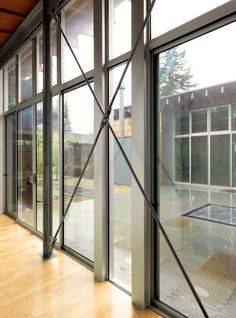# Truss problem with counter members

• Engineering
• mingyz0403
In summary, the conversation discusses solving a structural problem using the method of sections. It is explained that CD is a zero force member because it is redundant and can be removed without affecting the stability of the structure. The use of counter members in truss structures is also mentioned as a way to prevent deformation and damage in case of unexpected loads.

#### mingyz0403

Homework Statement
the diagonal members in the center panels of the truss shown are very slender and can act only in tension; such members are known as counters. determine the force in member de and in the counters that are acting under the given loading.
Relevant Equations
3 reaction equation.
Since the question only asked for one member, I was trying to solve it using method of section. I cut in the middle of BD. I was unable to solve it because it has 4 unknown. Then I looked at the solution, it say that CD is zero force member. I don’t understand why CD is zero force member. Could anyone explain what makes CD a zero force member.

#### Attachments

•16A65114-ED59-4892-8B4A-7426DED7D40D.png
185.9 KB · Views: 359
•Lnewqban
First note that there are no joints at the centres of the diagonal members so this is a simply pin jointed structure which means that all forces can only be in the direction of the members.

Your calculation of the reaction forces by taking moments is correct - reaction at A is 12 and at H is 15.

Simple trusses can easily be analysed by looking at a single point and calculating its forces.

Look at point A and sum the forces. There is a vertical force of 12 up at A, a force applied by the link AB and a force applied by the link AC. Equate the horizontal and vertical components to calculate the link forces. You now have the magnitude and direction of forces in members AB and AC. These forces are applied to the pivots B and C.

Now sum the forces in triangle ABC to get the force in BC.

Continue in this manner until you have calculated the forces in all members.

Why is CD a zero force member?

Take AC away - does the truss collapse? Why?

Now take CD away instead - does the truss collapse? Do you see the difference? CD is redundant - you only need triangles in a truss. If you can take a member away and the truss does not collapse that member is redundant.

Google analyse truss for lots of help.

Last edited:
•mingyz0403 and Lnewqban
mingyz0403 said:
... I don’t understand why CD is zero force member. Could anyone explain what makes CD a zero force member.
The above post is excellent.
Imagine that the counters are elastic and can stretch some.
Due to the shown loads, member DE will naturally tend to get lower than members BC and FG.
That will impose tension loads for counters BE and EF, which will keep member DE from relocating itself lower and deforming rectangles DBCE and DFGE.
Counters CD and DG are doing nothing to restraing member DE in place and could be removed with no consequences.

In actual metal structures, that cross-crossing setup for counters is common, since direction of accidental loads is not always as foreseen.
If we imagine that the truss of our problem is a bridge, hurricane or tornado winds could eventually create lifting loads stronger than the shown loads, which could be able to try to lift member DE respect to the other, possibly causing damage to the road.
In such event, the normally unloaded counters CD and DG will work under tension loads, preventing any deformation of the structure and damage of the road.•mingyz0403